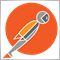# Counter not working in a large loop? Appreciate any help, thanks!7

Hi all,

Part of a script I am trying to run is needed to count the number of times that Price is equal to a value from an indicator.

the current function I have that I would expect to work is here:

```int GETCOUNT(double P)
{
int bar = 0;
int index = 0;

while (bar <= Bars)
{
if (iCustom(NULL,0,"ZigZag",12,5,3,0,bar) != 0)
{
if (P==iCustom(NULL,0,"ZigZag",12,5,3,0,bar))
{
index++;
}
}
bar++;
}
return(index);
}```

For some reason when I check the value of index it is always the same regardless of the price (P) that is passed to it. I have this function in a loop so that each price is checked through the function. Each price always hyas the same  number of Counts (index).

Sometimes the number will be 400 something, othertimes 3600 something and sometimes 0, but it always returns the same number of counts for each price.

Would really appreciate some help as I've been struggling for a few days to understand what could be wrong.2392

Never compare doubles using the equality operator because they are almost never equal. Instead you'll need to use CompareDoubles in stdlib or something similar.

```#include <stdlib.mqh>
int get_count(double price)
{
int count = 0;
for(int i=0; i<Bars; i++) {
double zigzag = iCustom(NULL,0,"ZigZag",12,5,3,0,i);
if(   zigzag != 0.0
&& zigzag == EMPTY_VALUE
&& CompareDoubles(price, zigzag)
){
count++;
}
}
return count;
}```